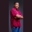Related Tags

javascript
communitycreator

# How to find the sum of even numbers in an interval in JavaScriptTheodore Kelechukwu Onyejiaku

Even numbers are numbers that leave no remainder when divided by two.

### Sum of even numbers in JavaScript

The sum of even numbers can be found using loops. We can use the for loop, while loop or do-while loop.

#### The trick

The trick to finding an even number is using the modulus%. The modulus and 2 will help to check if the number is even. See example below:

 //anynumber % 2

4 % 2 // = 0 (even number)
12 % 2 // = 0 (even number)

7 % 2 // = 1 (not an even number)
9 % 2 // = 1 (not an even number)


### Sum of even numbers using for loop

limit = 10;
sum = 0;

for(let i = 1; i <= 10; i++){
if(i % 2 == 0){
sum = sum + i;
}
}
console.log(The sum of even numbers from 0 - ${limit} is: \n${sum});

From the code above, we created the limit variable, which will tell us the limit of numbers we want or the limit of our interval. The sum is set to zero. For every loop, if the number is even, then it will be added to the sum.

### Sum of even numbers using while loop

In the code below, we created a counter that will keep the loop and serve as the numbers in the interval. We also created the sum and limit for the same reason as the one explained earlier. As the program loops, if the counter is even, it will be added to the sum.

let counter = 1;
let limit = 20;
let sum = 0;
while(counter <= limit){
if(counter % 2 == 0){
sum = sum + counter;
}
counter++;
}
console.log(The sum of even numbers from 0 - ${limit} is: \n${sum});

### Sum of even numbers using do-while loop

We can also use the do-while loop to achieve this purpose. It is the same code as the while loop. The only difference here is that it is a do-while loop.

let counter = 1;
let limit = 100;
let sum = 0;
do{
if(counter % 2 == 0){
sum = sum + counter;
}
counter++
}while(counter <= limit)

console.log(The sum of even numbers from 0 - ${limit} is: \n${sum});

RELATED TAGS

javascript
communitycreator

CONTRIBUTORTheodore Kelechukwu Onyejiaku
RELATED COURSES

View all Courses

Keep Exploring

Learn in-demand tech skills in half the time